# R S Aggarwal Solutions for Class 10 Maths Exercise 18A Chapter 18 Mean, Median, Mode of Grouped Data, Cumulative Frequency Graph and Ogive

R S Aggarwal Solutions for Class 10 Maths is one of the best study materials prepared by experienced subject experts. All questions are provided along with the answers for a better understanding of the concepts. This exercise is based on the mean of grouped data using different methods like direct method, assumed method and step derivation. Students can download Class 10 Maths Chapter 18 R S Aggarwal Solutions and start practicing offline.

## Download PDF of R S Aggarwal Solutions for Class 10 Maths Chapter 18 Mean, Median, Mode of Grouped Data, Cumulative Frequency Graph and Ogive Exercise 18A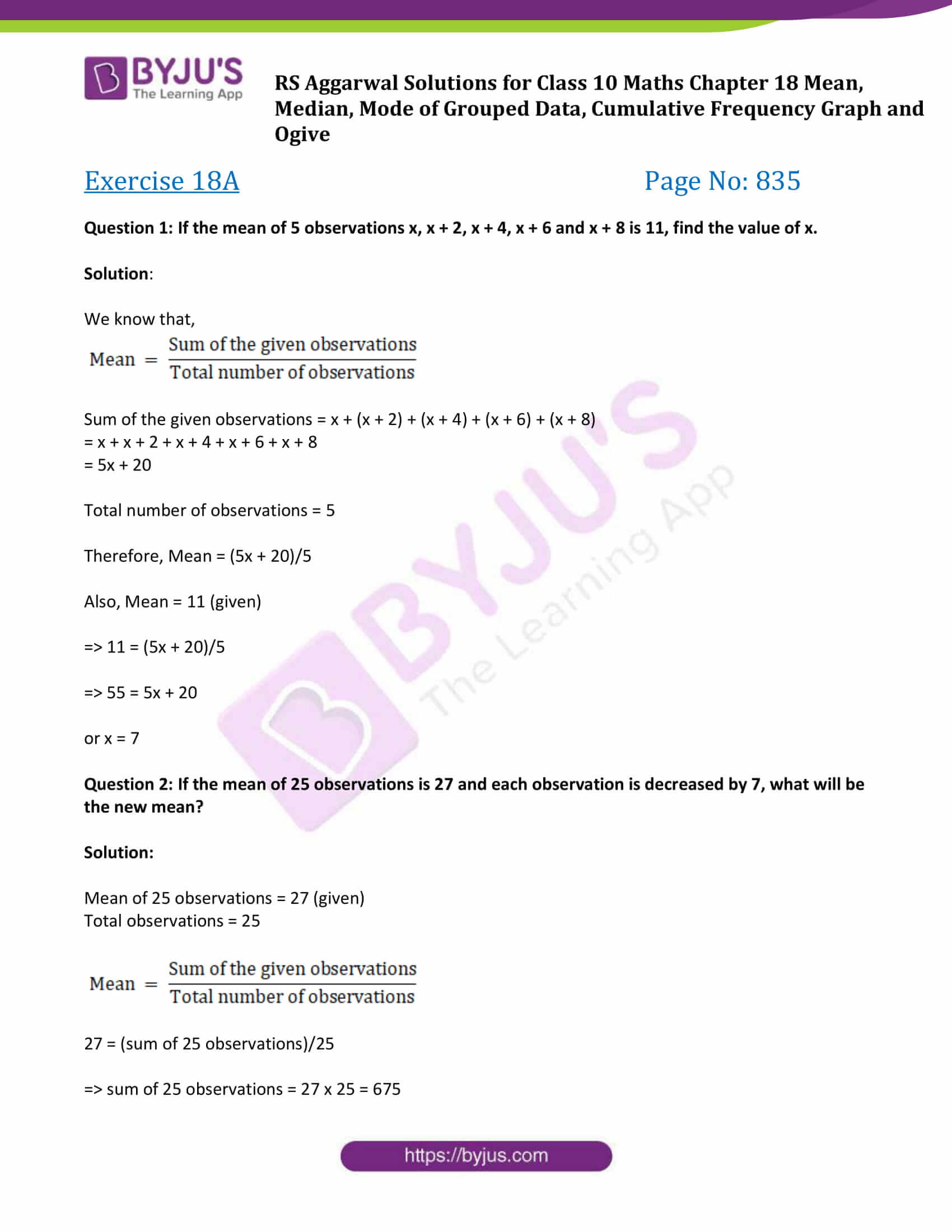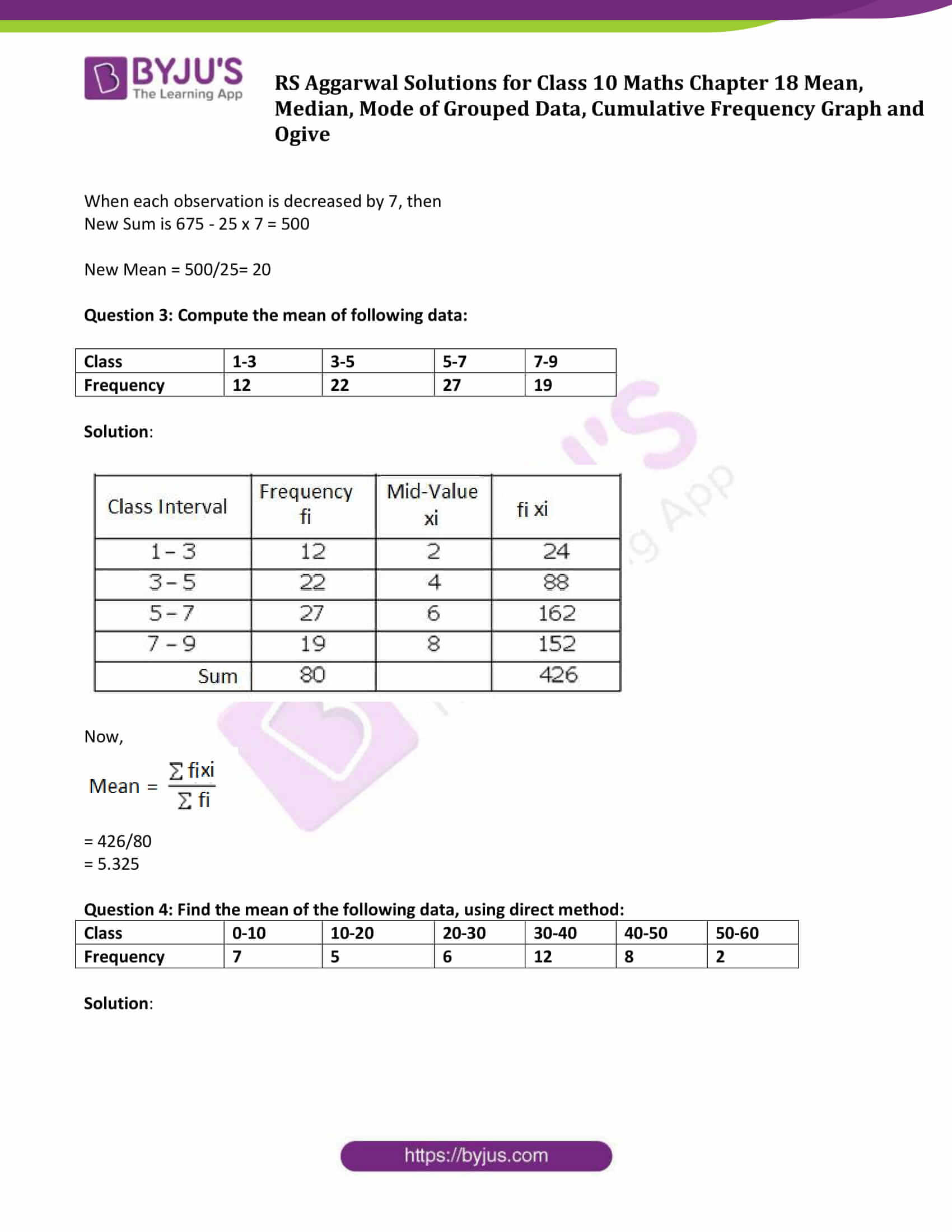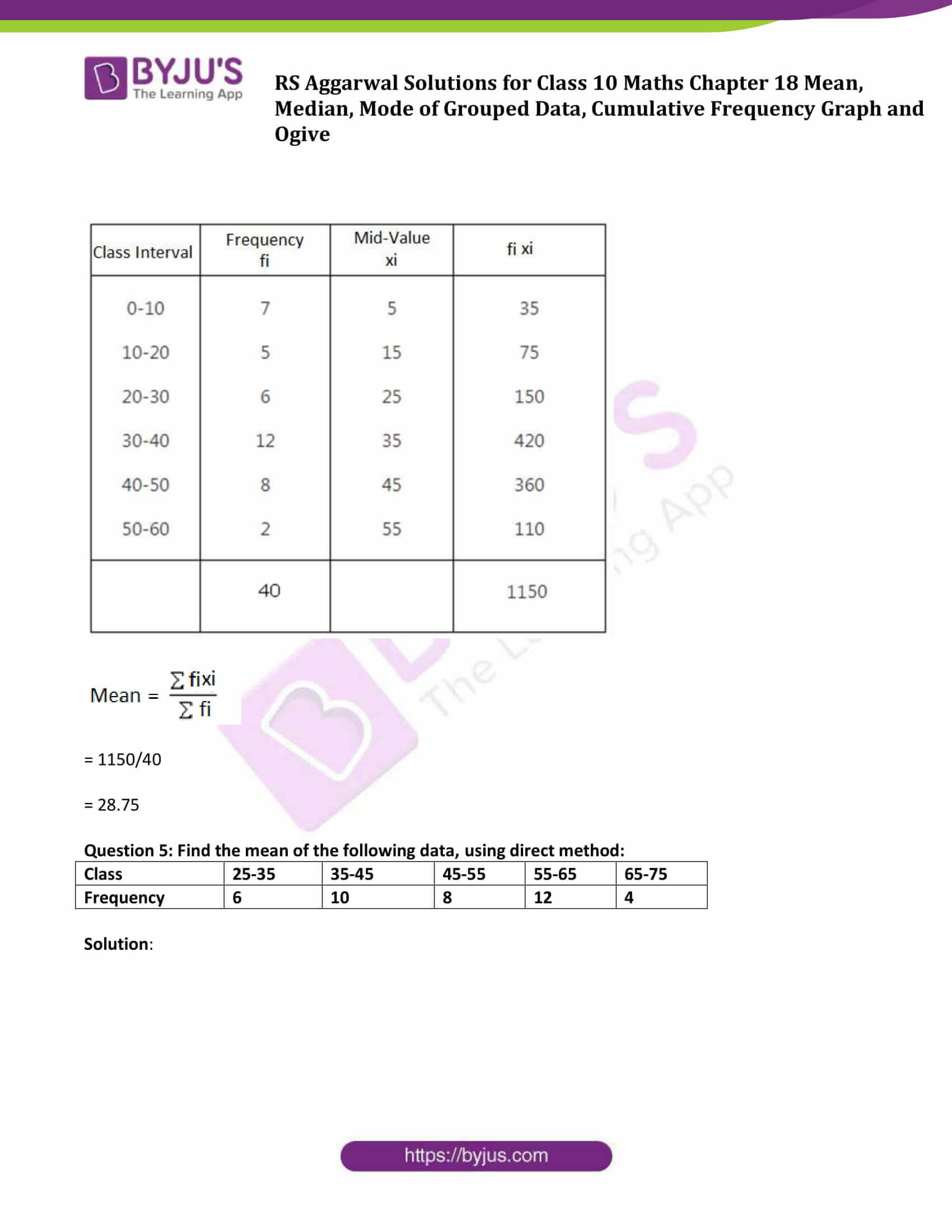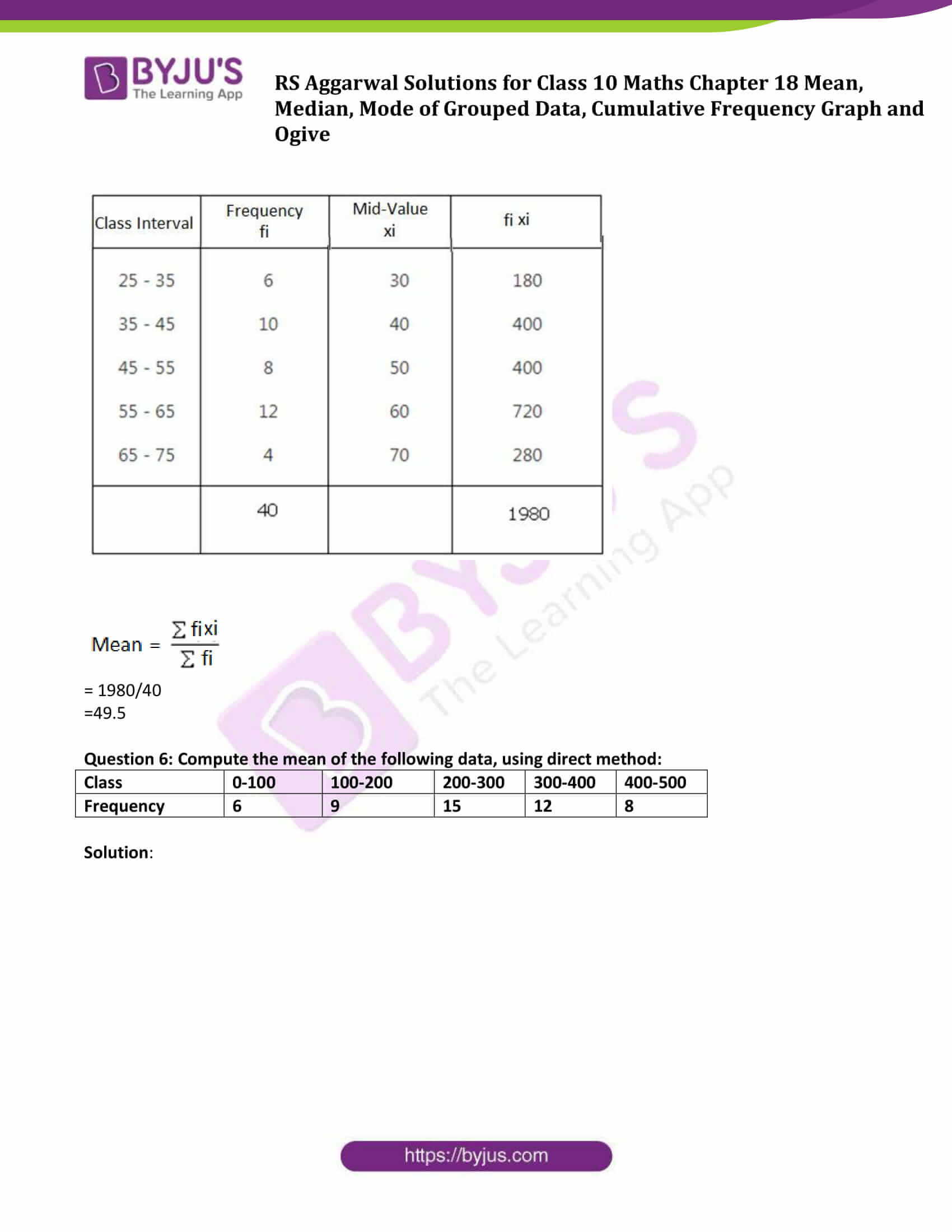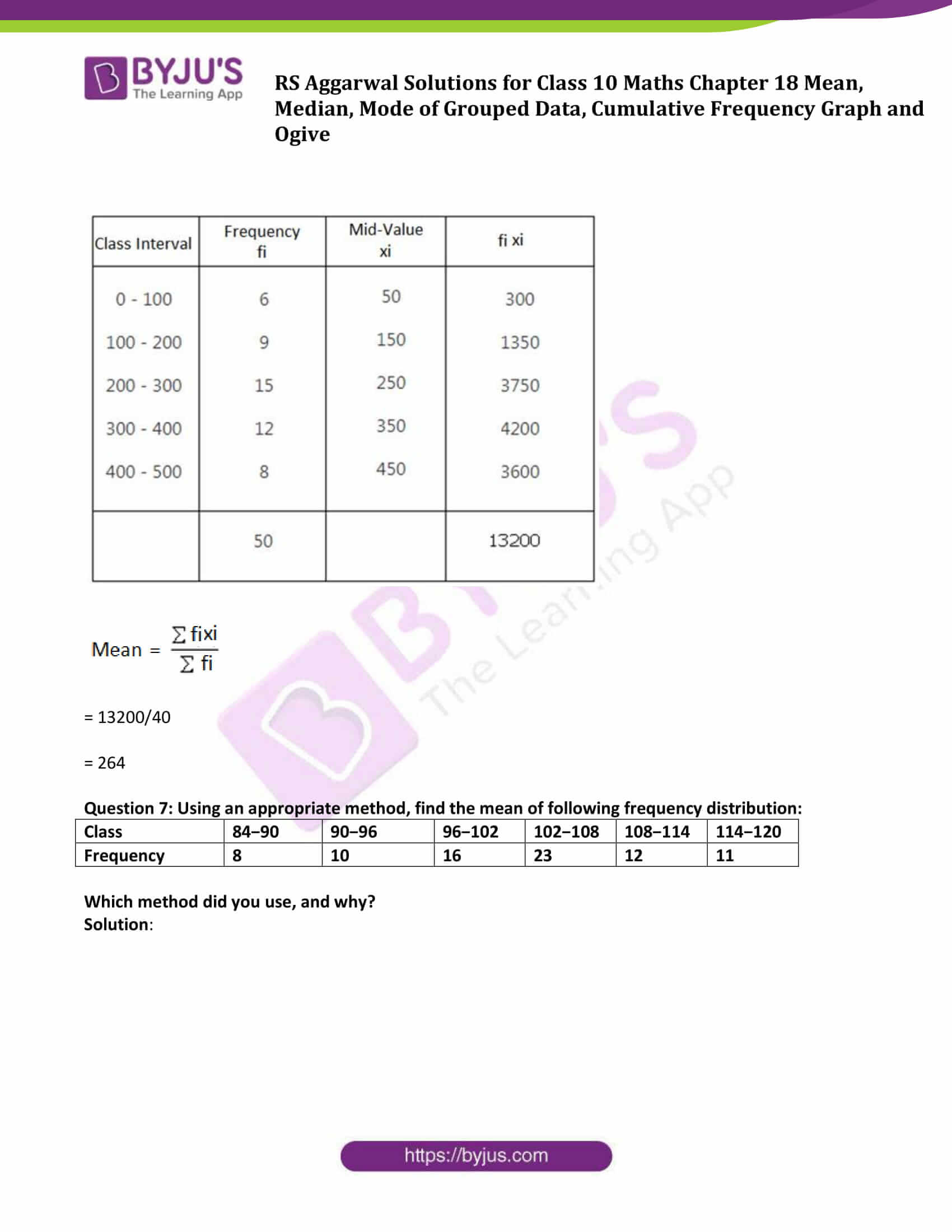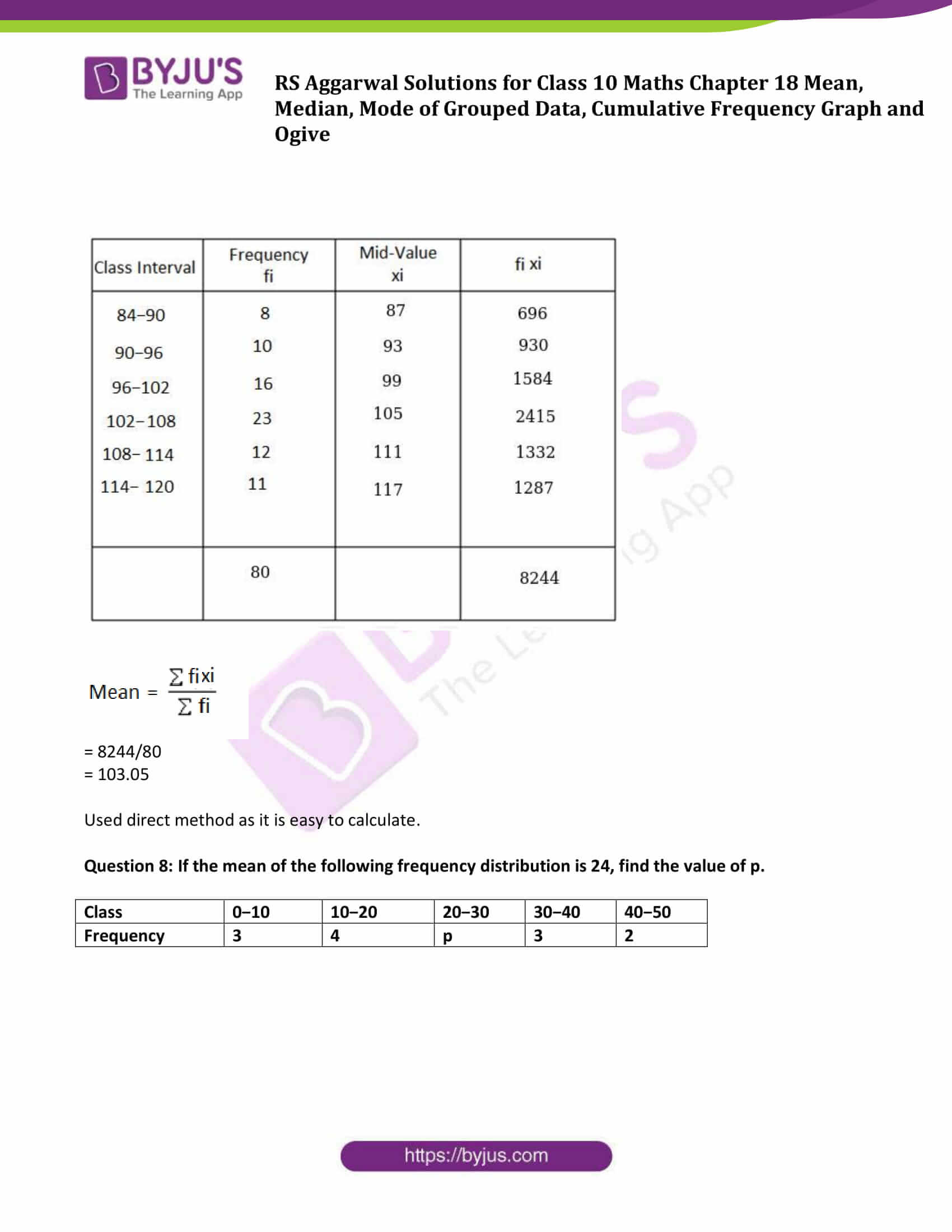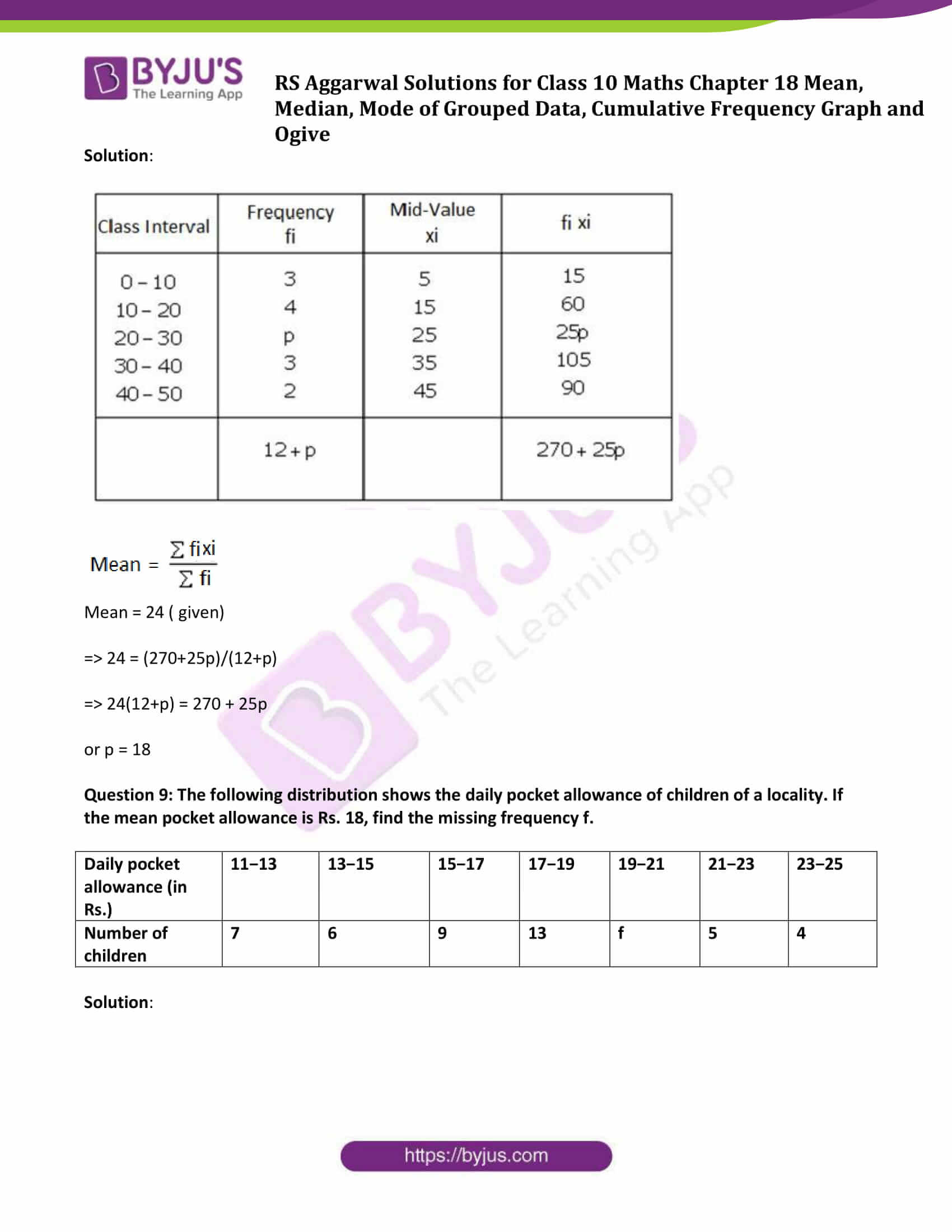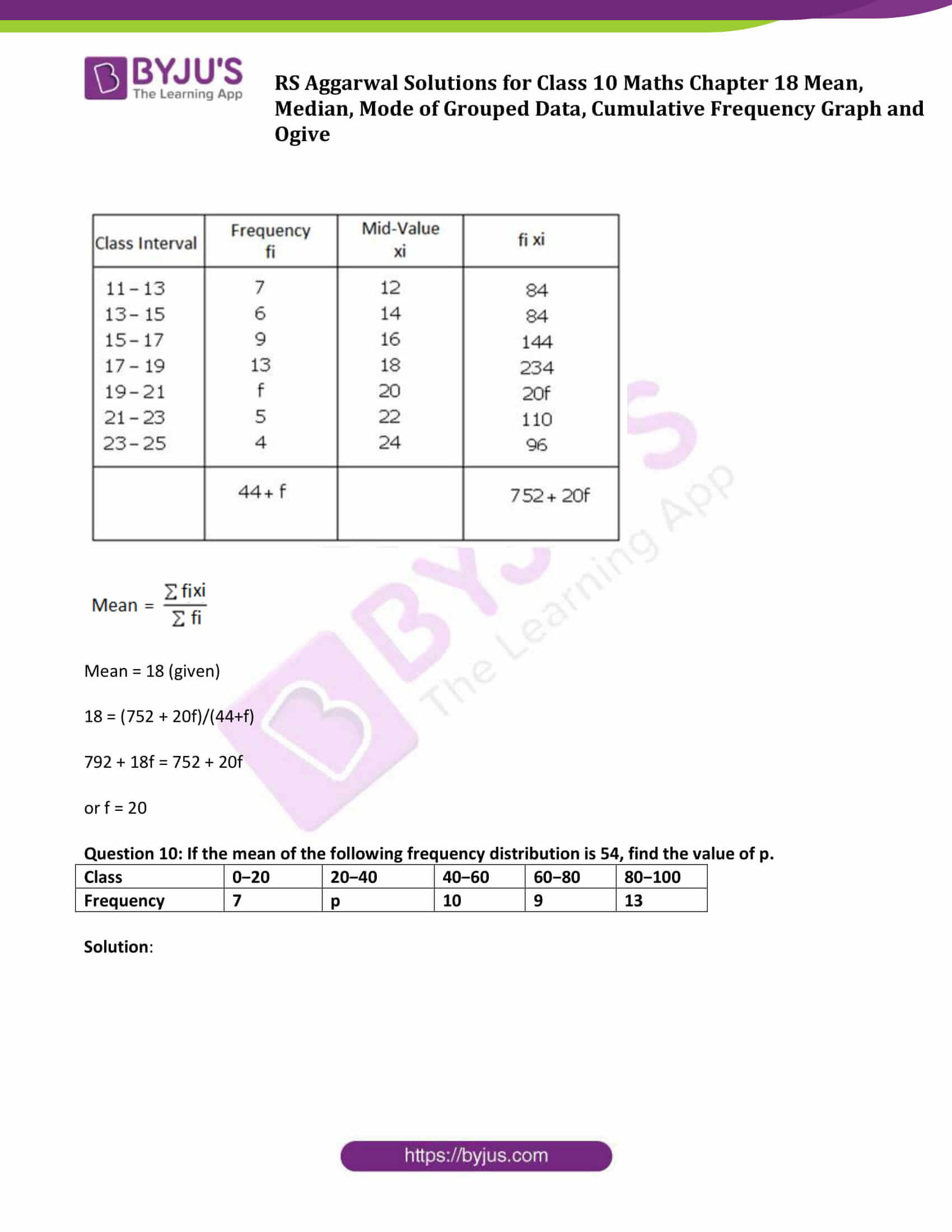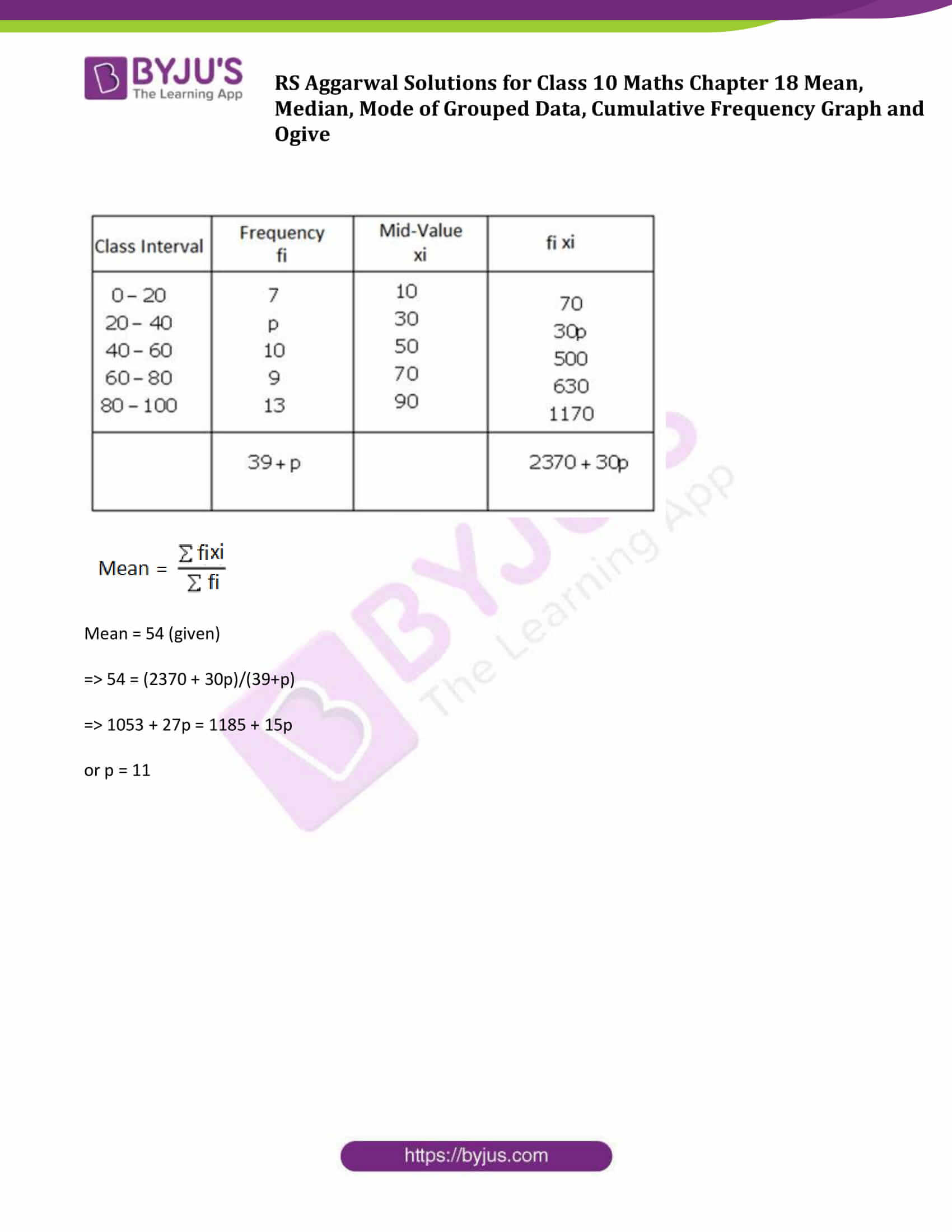## Access other exercise solutions of Class 10 Maths Chapter 18 Mean, Median, Mode of Grouped Data, Cumulative Frequency Graph and Ogive

Exercise 18 B Solutions

Exercise 18 C Solutions

Exercise 18 D Solutions

Exercise 18 E Solutions

### Access Solutions to Maths R S Aggarwal Class 10 Chapter 18 – Mean, Median, Mode of Grouped Data, Cumulative Frequency Graph and Ogive Exercise 18A

Question 1: If the mean of 5 observations x, x + 2, x + 4, x + 6 and x + 8 is 11, find the value of x.

Solution:

We know that,

$Mean = \frac{Sum\,of\, the\, given\, observations}{Total\, number\, of\, observations}$

Sum of the given observations = x + (x + 2) + (x + 4) + (x + 6) + (x + 8)

= x + x + 2 + x + 4 + x + 6 + x + 8

= 5x + 20

Total number of observations = 5

Therefore, Mean = (5x + 20)/5

Also, Mean = 11 (given)

⇨ 11 = (5x + 20)/5

⇨ 55 = 5x + 20

or x = 7

Question 2: If the mean of 25 observations is 27 and each observation is decreased by 7, what will be the new mean?

Solution:

Mean of 25 observations = 27 (given)

Total observations = 25

$Mean = \frac{Sum\,of\, the\, given\, observations}{Total\, number\, of\, observations}$

27 = (sum of 25 observations)/25

⇨ sum of 25 observations = 27 x 25 = 675

When each observation is decreased by 7, then

New Sum is 675 – 25 x 7 = 500

New Mean = 500/25= 20

Question 3: Compute the mean of following data:

 Class 1-3 3-5 5-7 7-9 Frequency 12 22 27 19

Solution: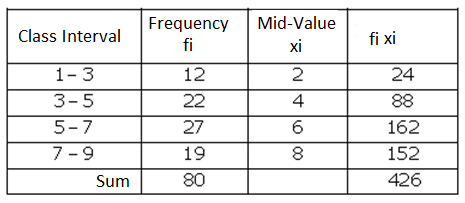Now,

$Mean = \frac{\sum fixi}{\sum fi}$

= 426/80

= 5.325

Question 4: Find the mean of the following data, using direct method:

 Class 0-10 10-20 20-30 30-40 40-50 50-60 Frequency 7 5 6 12 8 2

Solution: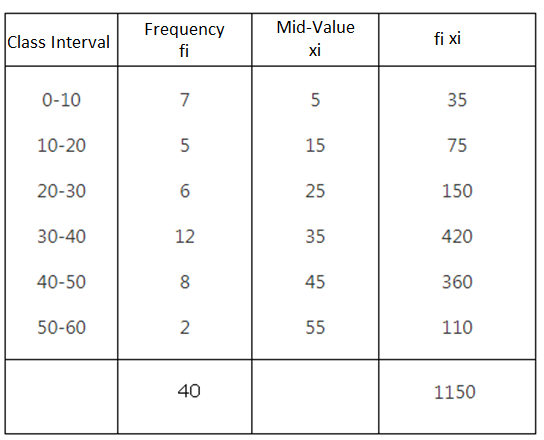$Mean = \frac{\sum fixi}{\sum fi}$

= 1150/40

= 28.75

Question 5: Find the mean of the following data, using direct method:

 Class 25-35 35-45 45-55 55-65 65-75 Frequency 6 10 8 12 4

Solution: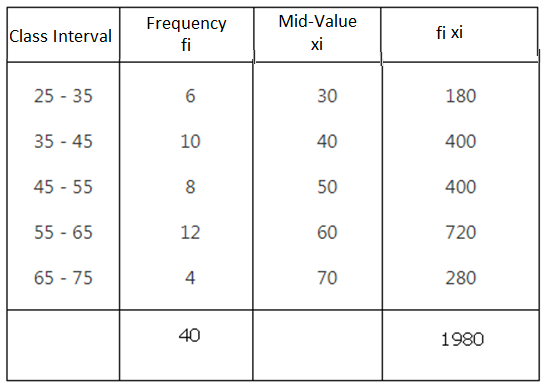$Mean = \frac{\sum fixi}{\sum fi}$

= 1980/40

=49.5

Question 6: Compute the mean of the following data, using direct method:

 Class 0-100 100-200 200-300 300-400 400-500 Frequency 6 9 15 12 8

Solution: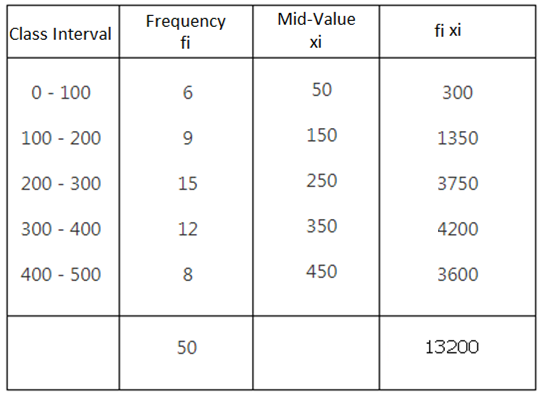$Mean = \frac{\sum fixi}{\sum fi}$

= 13200/40

= 264

Question 7: Using an appropriate method, find the mean of following frequency distribution:

 Class 84−90 90−96 96−102 102−108 108−114 114−120 Frequency 8 10 16 23 12 11

Which method did you use, and why?

Solution: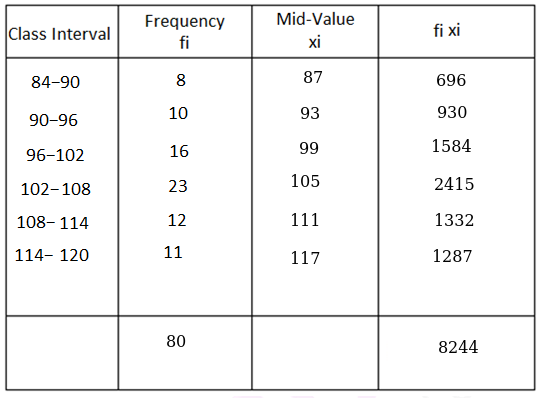$Mean = \frac{\sum fixi}{\sum fi}$

= 8244/80

= 103.05

Used direct method as it is easy to calculate.

Question 8: If the mean of the following frequency distribution is 24, find the value of p.

 Class 0−10 10−20 20−30 30−40 40−50 Frequency 3 4 p 3 2

Solution: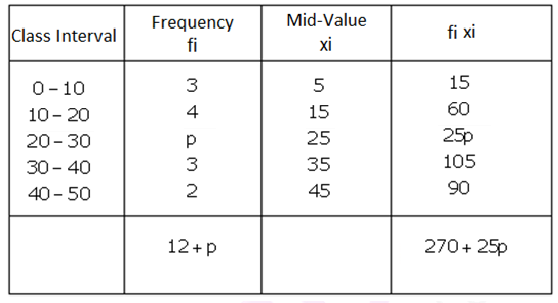$Mean = \frac{\sum fixi}{\sum fi}$

Mean = 24 ( given)

⇨ 24 = (270+25p)/(12+p)

⇨ 24(12+p) = 270 + 25p

or p = 18

Question 9: The following distribution shows the daily pocket allowance of children of a locality. If the mean pocket allowance is Rs. 18, find the missing frequency f.

 Daily pocket allowance (in Rs.) 11−13 13−15 15−17 17−19 19−21 21−23 23−25 Number of children 7 6 9 13 f 5 4

Solution: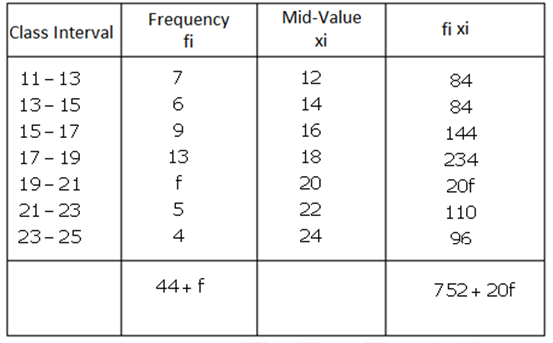$Mean = \frac{\sum fixi}{\sum fi}$

Mean = 18 (given)

18 = (752 + 20f)/(44+f)

792 + 18f = 752 + 20f

or f = 20

Question 10: If the mean of the following frequency distribution is 54, find the value of p.

 Class 0−20 20−40 40−60 60−80 80−100 Frequency 7 p 10 9 13

Solution: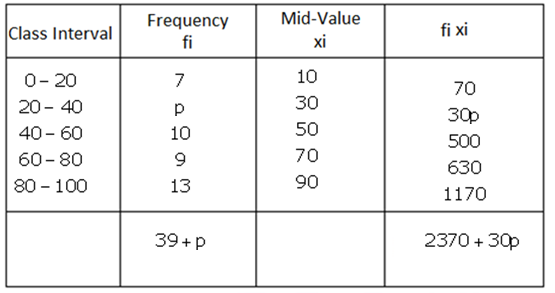$Mean = \frac{\sum fixi}{\sum fi}$

Mean = 54 (given)

⇨ 54 = (2370 + 30p)/(39+p)

⇨ 1053 + 27p = 1185 + 15p

or p = 11

## R S Aggarwal Solutions for Class 10 Maths Chapter 18 Mean, Median, Mode of Grouped Data, Cumulative Frequency Graph and Ogive Exercise 18A Topics:

Class 10 Maths Chapter 18 Mean, Median, Mode of Grouped Data, Cumulative Frequency Graph and Ogive Exercise 18A is based on the following topics:

• Mean of grouped data – Direct Method
• Mean of grouped data – Assumed-Mean Method
• Mean of grouped data – Step derivation method
• Mathematical derivation of assumed mean formula
• Mathematical derivation of step derivation method
• Mean for an inclusive series